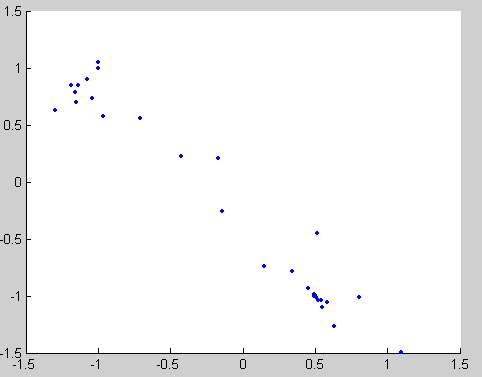## 优化求解器输出函数

### 什么是输出函数？

`OutputFcn` 选项可以与下列 MATLAB® 优化函数配合使用：

### 创建和使用输出函数

```function stop = outfun(x, optimValues, state) stop = false; hold on; plot(x(1),x(2),'.'); drawnow```

`$\underset{x}{\mathrm{min}}f\left(x\right)=\underset{x}{\mathrm{min}}{e}^{{x}_{1}}\left(4{x}_{1}^{2}+2{x}_{2}^{2}+{x}_{1}{x}_{2}+2{x}_{2}\right).$`

1. 创建一个包含前述代码的文件，并将其作为 `outfun.m` 保存在 MATLAB 路径的文件夹中。

2. `options` 结构体的 `Outputfcn` 字段的值设置为 `outfun` 的函数句柄。

`options = optimset('OutputFcn', @outfun);`
3. 输入以下命令：

```hold on objfun=@(x) exp(x(1))*(4*x(1)^2+2*x(2)^2+x(1)*x(2)+2*x(2)); [x fval] = fminsearch(objfun, [-1 1], options) hold off```

这些命令返回解

```x = 0.1290 -0.5323 fval = -0.5689```

并显示以下关于 `fminsearch` 生成的点的绘图：### 输出函数的结构体

`stop = outfun(x, optimValues, state)`

• `stop` 是一个标志，根据优化例程是停止还是继续，该标志为 `true``false`。请参阅 Stop 标签

• `x` 是算法在当前迭代中计算的点。

• `optimValues` 是包含当前迭代中的数据的结构体。optimValues 中的字段详细介绍了此结构体。

• `state` 是算法的当前状态。算法的状态列出了可能的值。

### 嵌套输出函数的示例

• 以嵌套函数的形式包括输出函数 - 有关详细信息，请参阅 MATLAB 编程基础中的嵌套函数

• 调用优化函数。

`$\underset{x}{\mathrm{min}}f\left(x\right)=\underset{x}{\mathrm{min}}{e}^{{x}_{1}}\left(4{x}_{1}^{2}+2{x}_{2}^{2}+{x}_{1}{x}_{2}+2{x}_{2}\right).$`

1. 使用 MATLAB 编辑器打开一个新文件。

2. 将以下代码复制并粘贴到此文件。

```function [x fval history] = myproblem(x0) history = []; options = optimset('OutputFcn', @myoutput); [x fval] = fminsearch(@objfun, x0,options); function stop = myoutput(x,optimvalues,state); stop = false; if isequal(state,'iter') history = [history; x]; end end function z = objfun(x) z = exp(x(1))*(4*x(1)^2+2*x(2)^2+x(1)*x(2)+2*x(2)); end end```
3. 将文件作为 `myproblem.m` 保存到 MATLAB 路径上的某个文件夹中。

4. 在 MATLAB 提示符下，输入

`[x fval history] = myproblem([-1 1]);`

```x,fval x = 0.1290 -0.5323 fval = -0.5689```

```history(1:4,:) ans = -1.0000 1.0000 -1.0000 1.0000 -1.0750 0.9000 -1.0125 0.8500```

`history` 最后一行的点与最佳点 `x` 相同。

```history(end,:) ans = 0.1290 -0.5323 objfun(history(end,:)) ans = -0.5689```

### optimValues 中的字段

optimValues 字段 (optimValues.field)

`funccount`

`Func-count`

`fval`

`min f(x)`

`iteration`

`Iteration`

`procedure`

`Procedure`

### 算法的状态

`'init'`

`'interrupt'`

`'iter'`

`'done'`

```switch state case 'init' % Setup for plots or dialog boxes case 'iter' % Make updates to plots or dialog boxes as needed case 'interrupt' % Check conditions to see whether optimization % should quit case 'done' % Cleanup of plots, dialog boxes, or final plot end```

### Stop 标签

#### 根据 optimValues 中的数据停止优化

```function stop = myoutput(x, optimValues, state) stop = false; % Check if objective function is less than 5. if optimValues.fval < 5 stop = true; end```

#### 基于对话框输入停止优化

```function stop = myoutput(x, optimValues, state) stop = false; % Check if user has requested to stop the optimization. stop = getappdata(hObject,'optimstop');```